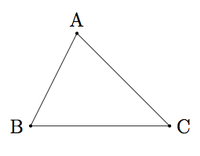# Circumcenter of a triangle

Alignments to Content Standards: G-C.A.3

The goal of this task is to construct the circumcenter of a triangle, that is, the point which is the same distance from each of the triangles three vertices. A sample triangle is pictured below:The collection of points equidistant from $B$ and $C$ is the perpendicular bisector $\ell$ of $\overline{BC}$ pictured below:The collection of points equidistant from $A$ and $C$ is the perpendicular bisector $m$ of $\overline{AC}$, and we let $O$ be the point of intersection of $\ell$ and $m$, displayed below:Show that $O$ also lies on the perpendicular bisector of $\overline{AB}$.

## IM Commentary

This task shows that the three perpendicular bisectors of the sides of a triangle all meet in a point, using the characterization of the perpendicular bisector of a line segment as the set of points equidistant from the two ends of the segment. The point so constructed is called the circumcenter of the triangle. Note that whereas for the triangle drawn the circumcenter is on the interior of the triangle, the teacher may want to have students experiment with finding the circumcenter of different triangles. Ideally, they should be able to find examples where $O$ is on one of the sides of the triangle and also examples where $O$ is on the exterior of the triangle.

The circle with radius $|AO|$ and center $O$ contains all three vertices of $\triangle ABC$ and so this construction provides a way to inscribe a triangle in a circle. This is discussed further in ''Inscribing a triangle in a circle'' but the construction of the circumcenter is performed here. This task could appropriately used for assessment for the aforementioned characterization of the perpendicular bisector.

## Solution

Since $O$ lies on $\ell$, the perpendicular bisector of $\overline{BC}$, we know that $O$ is equidistant from $B$ and $C$, that is $$|OB| = |OC|.$$ Since $O$ lies on $m$, the perpendicular bisector of $\overline{AC}$, we know that $O$ is equidistant from $A$ and $C$, that is $$|OA| = |OC|.$$ Combining the two previous equalities we see that $\overline{OB}$ is congruent to $\overline{OA}$. This means that $O$ is equidistant from $A$ and $B$ and so $O$ also lies on the perpendicular bisector of $\overline{AB}$.